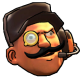## Posts Tagged ‘octagon’## How to draw a Perfect Octagon!

Tuesday, November 11th, 2014 4:45 am

I did some maths while I was bored, and I calculated a way to draw a perfect octagon.
I thought this might come in useful for some of you :3

Maths:

Imagine a square, with side length a.Our octagon will fit in this square.

We will call the length of a side of the octagon b.Now you put a into this equation:Which can also be written as:And you get b.

Now, round b to the nearest number. We can’t be dealing with fractions of pixels now, can we?

Now you have b, we can draw the octagon.

Drawing:

For this example, our reference point will be in the center of the octagon, which is also the center of our imaginary square.Our first 2 co-ordinates will be a/2 up and b/2 to either side.For the sides, it will be a/2 to the side and b/2 up or down.Then, draw lines between the co-ordinates to finish the octagon.And there you have a regular octagon!

Pseudo code:

Initialize:

size = (length of side of square)
oct_side = round((size*sqrt(2))/(2+sqrt(2)))

Loop:

coord_1x = x – oct_side/2
coord_1y = y + size/2
coord_2x = x + oct_side/2
coord_2y = y + size/2
coord_3x = x + size/2
coord_3y = y + oct_side/2
coord_4x = x + size/2
coord_4y = y – oct_side/2
coord_5x = x + oct_side/2
coord_5y = y – size/2
coord_6x = x – oct_side/2
coord_6y = y – size/2
coord_7x = x – size/2
coord_7y = y – oct_side/2
coord_8x = x – size/2
coord_8y = y + oct_side/2//draw_line(from_x,from_y,to_x,to_y)

draw_line(coord_1x,coord_1y,coord_2x,coord_2y)
draw_line(coord_2x,coord_2y,coord_3x,coord_3y)
draw_line(coord_3x,coord_3y,coord_4x,coord_4y)
draw_line(coord_4x,coord_4y,coord_5x,coord_5y)
draw_line(coord_5x,coord_5y,coord_6x,coord_6y)
draw_line(coord_6x,coord_6y,coord_7x,coord_7y)
draw_line(coord_7x,coord_7y,coord_8x,coord_8y)
draw_line(coord_8x,coord_8y,coord_1x,coord_1y)

And that’s how to draw a perfect octagon!

Coming soon: How to draw a perfect hexagon!

To those interested in the maths involved to get the equation:I originally calculated this to be able to draw the Aperture Science logo by hand, but I also saw the use of it on the computer!

[cache: storing page]